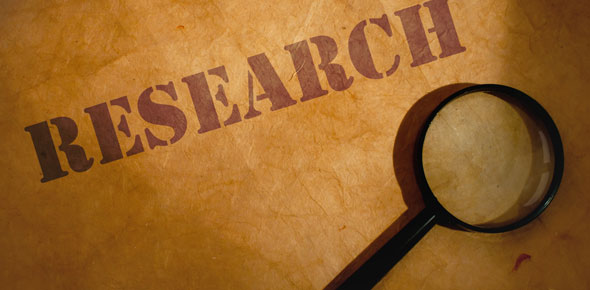# Psychological Research Methods II

15 Questions | Total Attempts: 55SettingsKnow correlation. Know simple regression. Know multiple regression. Know statistical regression with forward, backward, stepwise methods. Polytomous variables.

• 1.
The standard measure of the relationship between 2 variables is:
• A.

The standard deviation

• B.

The covariance

• C.

The coefficient of determination

• D.

The Pearson product-moment correlation coefficient

• 2.
If performance on 2 variables is related, it is:
• A.

Reciprocal

• B.

Relational

• C.

Correlated

• D.

None of the above

• 3.
Scatterplots:
• A.

Theorize a relationship

• B.

Provide a picture of the relationship between the 2 variables

• C.

Demonstrate causality

• D.

Represent measurement

• 4.
A lower left to upper right pattern in a scatterplot demonstrates:
• A.

Positive relationship or correlation

• B.

Negative relationship or correlation

• C.

Slight positive correlation

• D.

Slight negative correlation

• 5.
A perfect correlation is demonstrated by:
• A.

All points lie on straight line

• B.

Most points lie on straight line

• C.

Some points lie on straight line

• D.

None of the above

• 6.
The correlation coefficient :
• A.

Is the qualitative index of the r'ship

• B.

Doesn't describe the measurement between 2 variables

• C.

Similar to the correlation

• D.

Is the quantitative index of the r'ship

• 7.
The absolute value of the coefficient indicates the:
• A.

Magnitude of the relationship

• B.

Anything but the magnitude of the r'ship

• C.

None of the above

• 8.
A correlation soefficient of + .1 or - .1 indicates:
• A.

A high correlation

• B.

Low correlation

• C.

No correlation

• D.

Some corrrelation

• 9.
No relationship between the variables is:
• A.

Zero

• B.

One

• C.

Minus one

• D.

Plus one

• 10.
The slope:
• A.

Indicates the general direction of the relationship

• B.

Is always positive

• C.

Is always negative

• D.

None of the above

• 11.
The width of the ellipse indicates what of the correlation coefficient:
• A.

Extent of the r'ship

• B.

The magnitude

• C.

The absolute value

• D.

All of the above

• 12.
In essence, the product-moment correlation coefficient is:
• A.

The sum of the cross-products

• B.

The multiplication of cross-products

• C.

The end result of 2 variables

• D.

The mean of the cross-products of scores

• 13.
The coefficient, r, is:
• A.

The standardized scores

• B.

The raw scores

• C.

A combined result

• D.

None of the above

• 14.
The Pearson product-moment correlation coefficient is the:
• A.

Average cross-product of the raw scores of 2 variables.

• B.

Average cross-product of the standard scores of 2 variables.

• C.

Average summed scores of the standard scores of 2 variables

• D.

Average summed scores of the raw scores of 2 variables.

• 15.
Factors affecting the size of Pearson, r:
• A.

Paired observations and nominal scale

• B.

Paired observations and using an interval scale

• C.

Paired observations and an ordinal scale

• D.

Single observations and an interval scale

Related TopicsBack to top